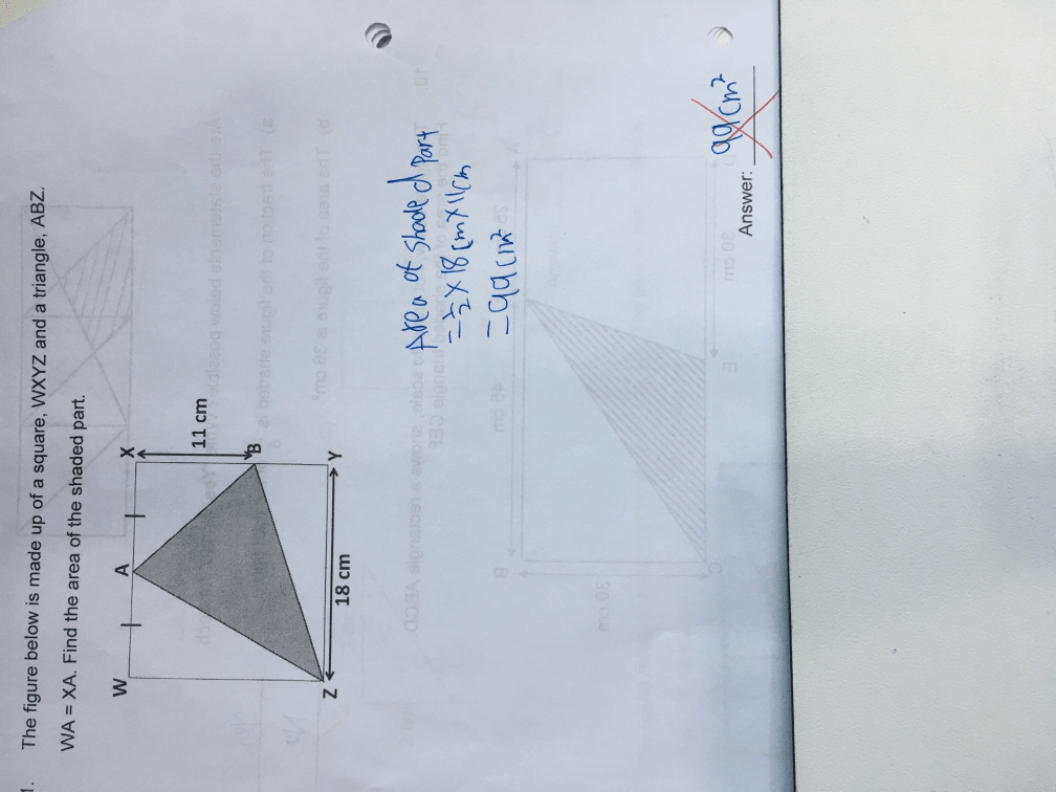# QuestionPls help.

Thanks.

1 Answer

# Answer

area of figure = area of square, WXYZ – area of triangles (AWZ + ABX + BYZ) = 18 x 18 – (1/2) x [(18/2) x 18 + (18/2) x 11 + 18 x (18 – 11) = 324 – 193.5 = 130.5 sq cm

Ans : 130.5 sq cm.

0 Replies 0 Likes